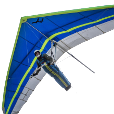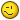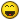# Need x and y values

## Recommended PostsIs there a quick and practical way to get X & Y values on a polyline other than by way of "LIST". because I need the X & Y value just like the 2nd image.

Thank you Master.

img 1 :

img 2 :

##### Share on other sitesYou could explode the polyline (so it becomes a LINE) and use EATTEXT to export the starting X and Y points of each line to an excel document. You would mis the last X and Y point though this way, so you need to add it manually or also select the end X and Y points and just delete what you don't need in excel (or some other spreadsheet program).

##### Share on other sitesJust add the write the answer out to this, the co-ords are in a list as (x y) for 2d plines or just use the getcords as a list of (x y x y x y)

```; pline co-ords example
; By Alan H
(defun getcoords (ent)
(vlax-safearray->list
(vlax-variant-value
(vlax-get-property
(vlax-ename->vla-object ent)
"Coordinates"
)
)
)
)

(defun co-ords2xy ()
; convert now to a list of xy as co-ords are x y x y x y if 3d x y z x y z
(setq numb (/ (length co-ords) 2))
(setq I 0)
(repeat numb
(setq xy (list (nth i co-ords)(nth (+ I 1) co-ords) ))
(setq co-ordsxy (cons xy co-ordsxy))
(setq I (+ I 2))
)
)

; program starts here
(setq co-ords (getcoords (car (entsel "\nplease pick pline"))))
(co-ords2xy)
; look at varaible co-ordsxy which is a list of vertices
(princ co-ordsxy)
```

##### Share on other sitesTry this:

```(defun c:foo ( / s )
(if (and (setq s (car (entsel "\nSelect a LWpolyline :")))
(member '(0 . "LWPOLYLINE") (setq s (entget s)))
)
(foreach pt s
(if (= (car pt) 10)
(mapcar 'princ (mapcar 'strcat '("\nX=" " Y=")  (mapcar '(lambda (x) (rtos x 2)) (cdr pt))))
)
)
(princ "\nNothing selected or invalid selection <!>")
)
(princ)
)
```

##### Share on other sitesHi, try this:

```; LWPOLYLINE's coordinates to excel
; Credits to: Lee Mac
(defun C:test ( / GroupN e xlapp xlwbs xlwbk xlsht xlcells xlrng msg )

(defun GroupN ( n L )
( (lambda (f) (f n L))
(lambda (n L) (cond ( (not L) L) ( (cons ((lambda ( / tmp ) (repeat n (setq tmp (cons (car L) tmp)) (setq L (cdr L))) (reverse tmp))) (f n L)) ) ) )
)
)

(cond
( (not (setq e (car (entsel "\nPick LWpolyline: ")))) (prompt "\nNo selection.") )
( (not (wcmatch (cdr (assoc 0 (entget e))) "LWPOLYLINE")) (prompt "\nInvalid object.") )
( (not (setq xlapp (vlax-get-or-create-object "Excel.Application"))) (prompt "\nUnable to interfere with Excel application.") )
( ; (apply 'strcat (mapcar 'chr '(87 114 105 116 116 101 110 32 98 121 32 71 114 114 114)))
(vl-catch-all-error-p
(setq msg
(vl-catch-all-apply
(function
(lambda ( / i )
(setq xlwbs (vlax-get-property xlapp 'Workbooks))
(setq xlsht (vlax-get-property  xlapp 'ActiveSheet))
(setq xlcells (vlax-get-property xlsht 'Cells))
(setq i 1)
(mapcar
(function
(lambda (x)
(vl-catch-all-apply 'vlax-put-property (list xlcells 'Item i 1 (car x)))
(vl-catch-all-apply 'vlax-put-property (list xlcells 'Item i 2 (cadr x)))
(setq i (1+ i))
)
)
(GroupN 2 (append (list "X" "Y") (mapcar '(lambda (x) (rtos x (getvar 'lunits) (getvar 'luprec))) (vlax-get (vlax-ename->vla-object e) 'Coordinates))))
); mapcar
(setq xlrng (vlax-get-property xlsht 'UsedRange))
(mapcar '(lambda (prp) (vl-catch-all-apply 'vlax-put-property (list xlrng prp -4108))) '(VerticalAlignment HorizontalAlignment)) ; xlCenter = -4108
(vlax-put-property xlapp 'Visible :vlax-true)
)
)
)
)
)
(prompt (strcat "\nError: " (vl-catch-all-error-message msg)))
)
); cond
(mapcar (function (lambda (x) (if (eq (type x) 'VLA-OBJECT) (vlax-release-object x)))) (list xlapp xlwbs xlwbk xlsht xlcells xlrng) )
(princ)
); defun C:test```

##### Share on other sitesYou could use a special VLISP program as noted above but if one is not available I would suggest you reconsider using the LIST command and do a copy-paste into Excel. In Excel click the Data tab and then the Text to Columns option and specify a space delimited file and you will have the x and y coordinates of the polyline in separate columns.

##### Share on other sitesTry this:

```(defun c:foo [/quote]

hi Tharwat please look at the 2nd image,

i think OP needs something without prefix "N=" or "E=" ?

[code]

(defun c:xytest (/ f fn i l ss)
;hanhphuc 18.09.2017
;test w/o *error*

(if
(and
(setq ss (ssget "_+.:S:E"'((0 . "LWPOLYLINE"))))
(setq i -1 l (vl-remove-if-not ''((x)(listp (cdr x)))(entget (ssname ss 0))))
(setq fn (strcat (getvar 'tempprefix)"XY.csv"))
(setq f (open fn "w")))
(progn
(write-line "X,Y" f)
(repeat (1-(length l))
;;;(print (vl-string-translate "() ""  ,"(vl-princ-to-string(cdr(nth i l)))) f)
(write-line (apply 'strcat (mapcar ''((x)(strcat (rtos x 2)",")) (cdr(nth (setq i (1+ i)) l)))) f)
)
(if f (close f))
(vl-cmdf "start" fn)[color="green"]; if default "*.csv" associated to EXCEL, but slow performance[/color]
);progn
)
(princ)
)
```

##### Share on other siteshi, try this:

` (apply 'strcat (mapcar 'chr '(87 114 105 116 116 101 110 32 98 121 32 71 114 114 114)))`

`(vl-list->string '( 76 79 76 ) )`

##### Share on other siteshi Tharwat please look at the 2nd image,

i think OP needs something without prefix "N=" or "E=" ?

Hi hanhphuc.

I wrote the codes for the first image HERE .##### Share on other sites`(vl-list->string '( 76 79 76 ) )`

That was just a humbly info, with no commercial purpose.Anyway I always forget about the vl-list->string function, more used with the (apply 'strcat (mapcar 'chr ...)) approach.

Not sure if OP wanted the output to excel, just thought to cover up my practice in this section of lisp.

##### Share on other sitesThat was just a humbly info, with no commercial purpose.Anyway I always forget about the vl-list->string function, more used with the (apply 'strcat (mapcar 'chr ...)) approach.

Not sure if OP wanted the output to excel, just thought to cover up my practice in this section of lisp.

nothing wrong you've provided a vanilla method, just curious the hidden message, it was creative##### Share on other sitesthanks all..

## Join the conversation

You can post now and register later. If you have an account, sign in now to post with your account.
Note: Your post will require moderator approval before it will be visible.×   Pasted as rich text.   Paste as plain text instead

Only 75 emoji are allowed.